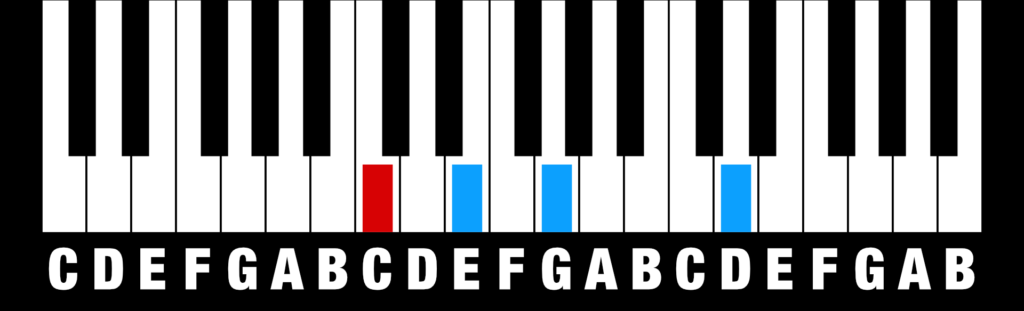# Learn (all) Piano Chords

Hello! My name is Mike, and I am a composer. Just. Like. You! =)

This is my complete list of all main chords that I personally use as a composer.

You can now learn and apply all these chords in your music, by using the formula of semi notes (half steps). Every chord formula below can be used on any root key.

R = Root key of the chord. The numbers that follow after “R” are the number of keys you need to step to the right to get to the next note in the chord.

PS. Make sure you bookmark this page, you are going to go back to it a lot! =)

### Major Triad (C) = R + 4 + 3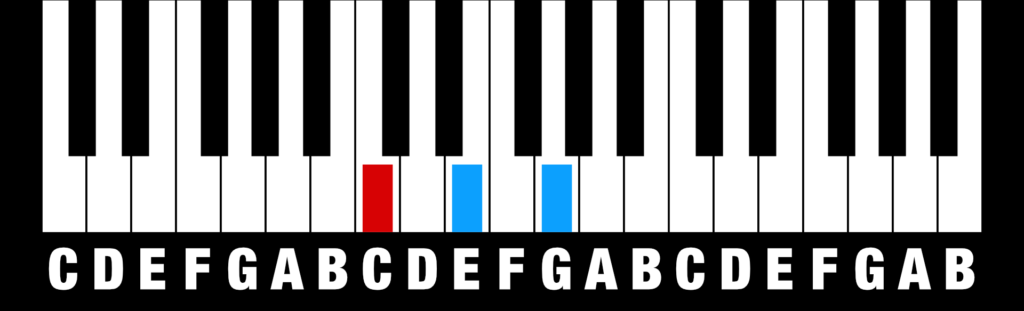### Minor Triad (Cm or Cmin)= R + 3 + 4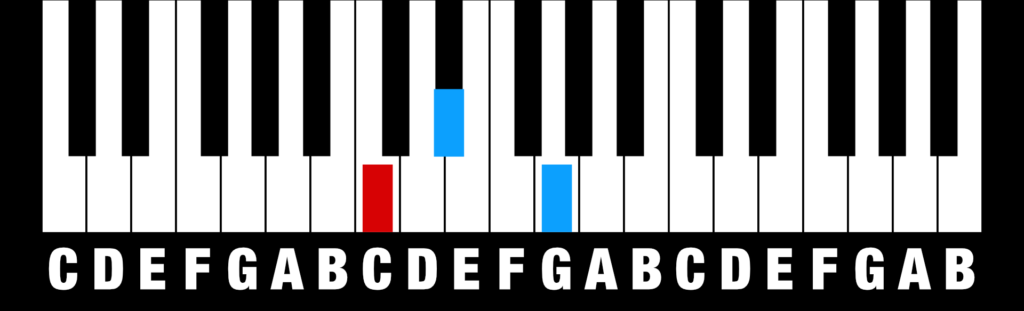### Diminished Triad (Co or Cdim) = R + 3 + 3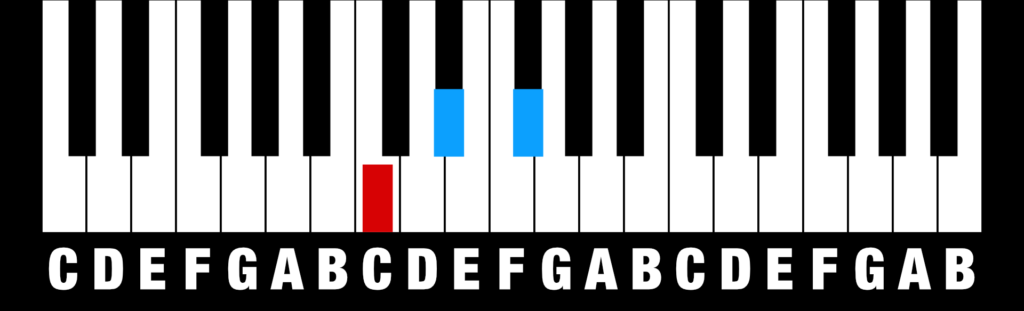### Augmented Triad (C+ or Caug) = R + 4 + 4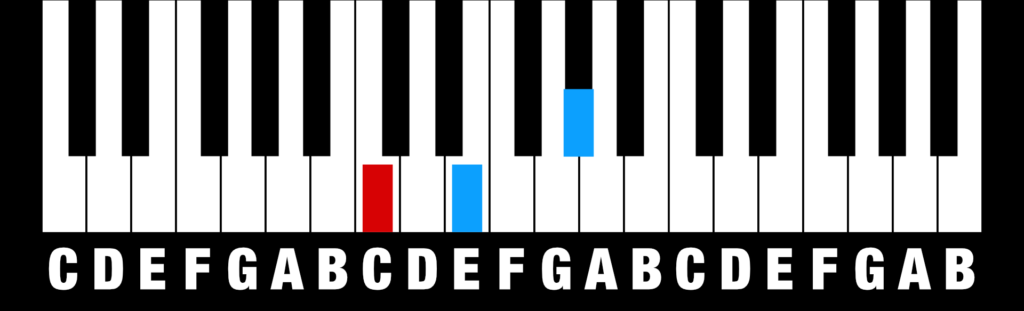### Suspended 2nd (Csus2) = R + 2 + 5### Suspended 4th (Csus4) = R + 5 + 2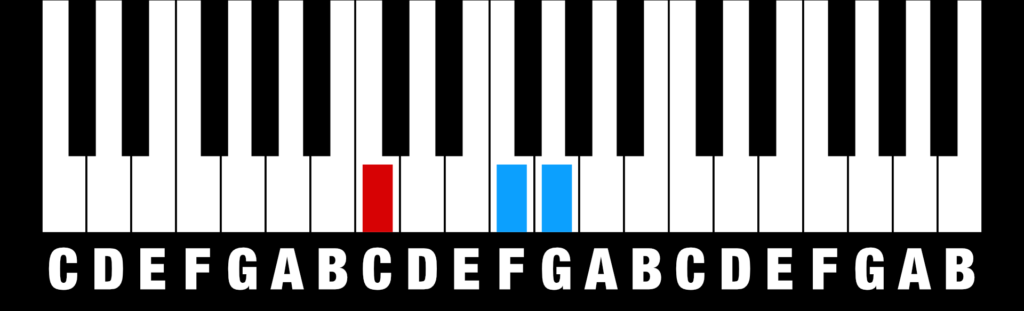### Major 6th (C6 or CM6 or Cmaj6) = R + 4 + 3 + 2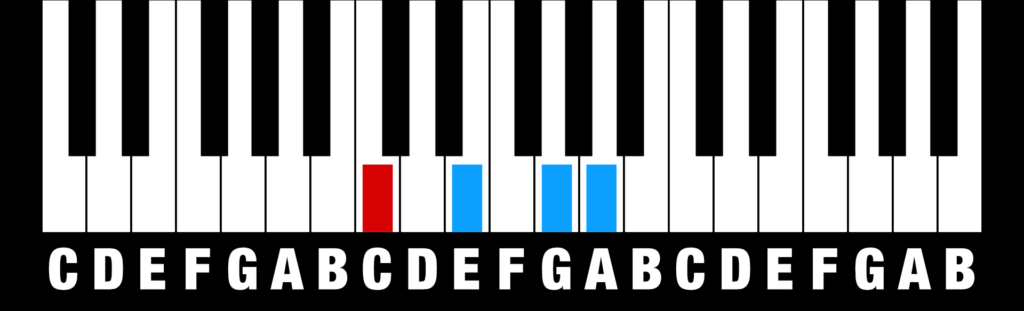### Minor 6th (Cm6 or Cmin6) = R + 3 + 4 + 2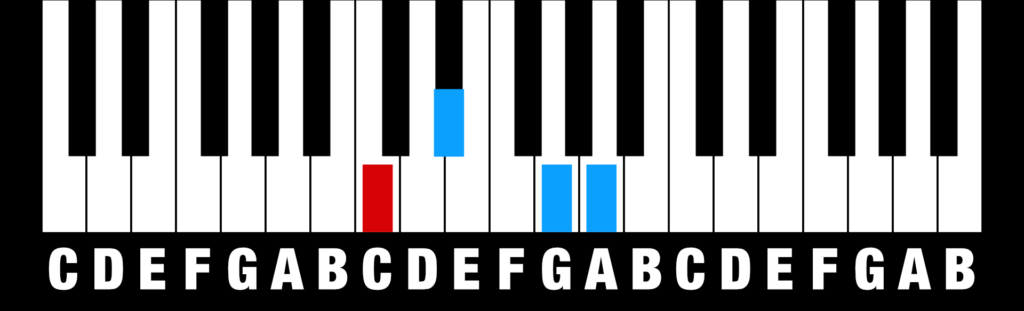### Dominant 7th (C7 or Cdom7) = R + 4 + 3 + 3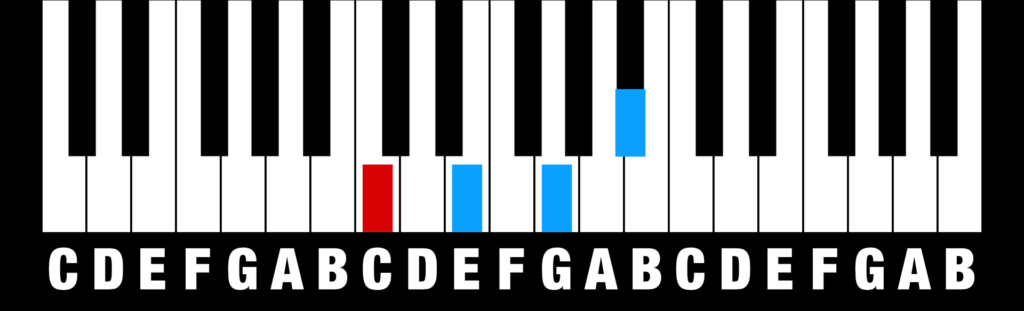### Major 7th (CM7 or Cmaj7) = R + 4 + 3 + 4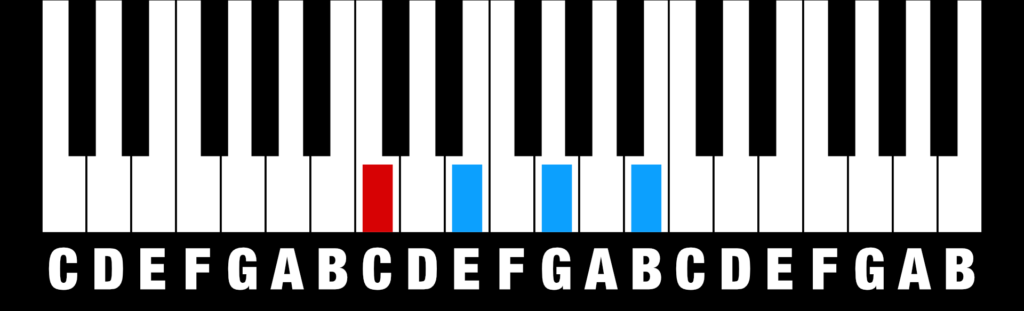### Minor 7th (Cm7 or Cmin7) = R + 3 + 4 + 3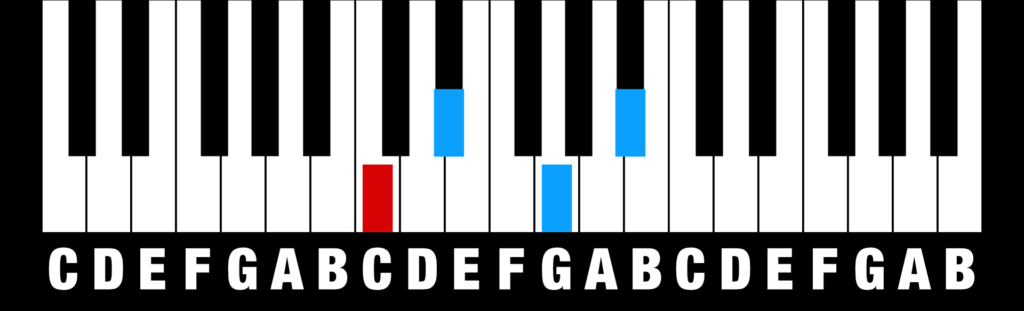### Minor-Major 7th (CmM7 or Cmin/maj7) = R + 3 + 4 + 4### Diminished 7th (Co7 or Cdim7) = R + 3 + 3 + 3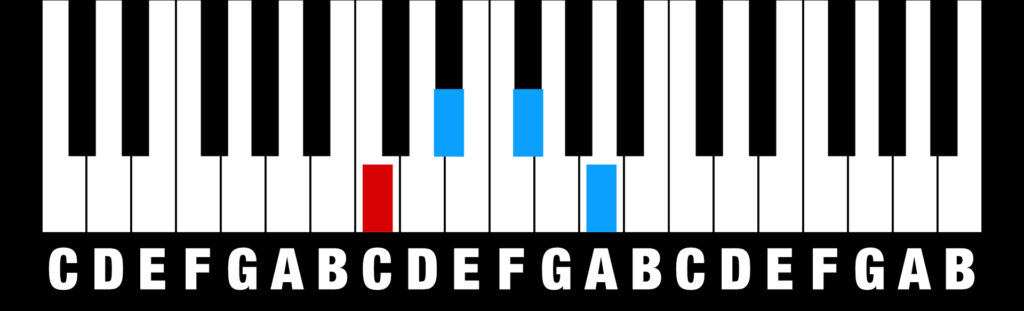### Half-Diminished 7th (Cø7 or Cm7♭5) = R + 3 + 3 + 4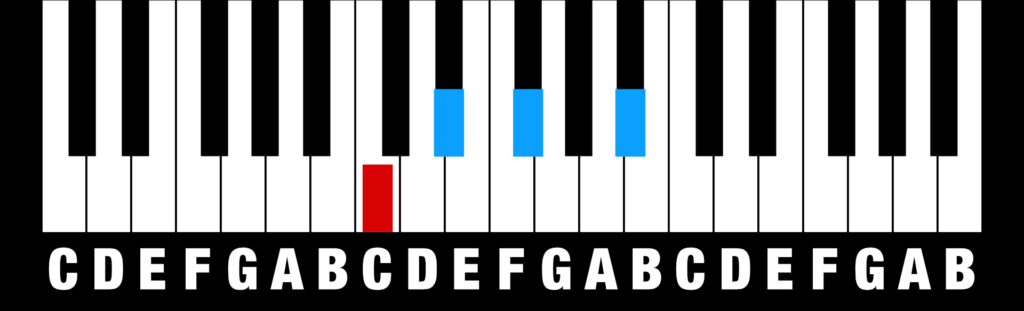### Augmented 7th (C+7 or Caug7 or C7♯5) = R + 4 + 4 + 2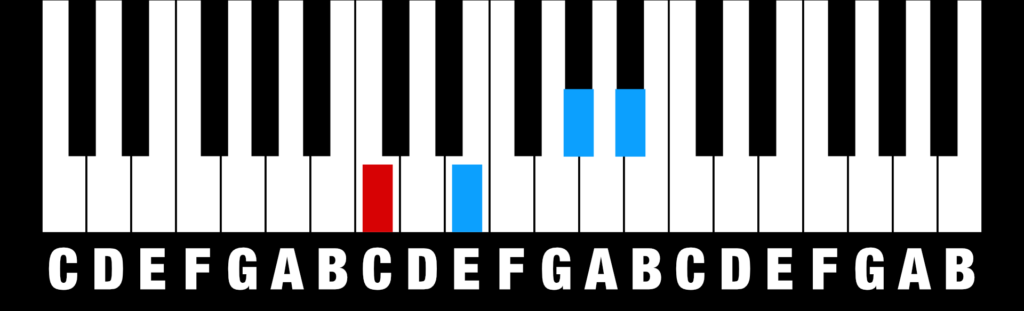### Augmented major 7th (C+M7 or CM7♯5) = R + 4 + 4 + 3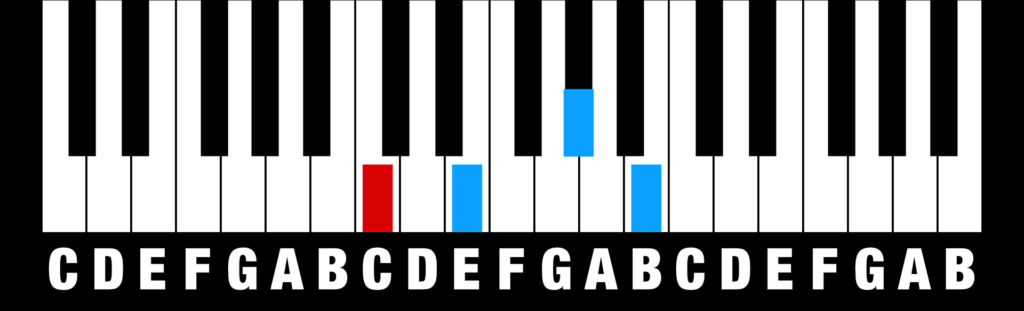### Dominant 9th (C9) = R + 4 + 3 + 3 + 4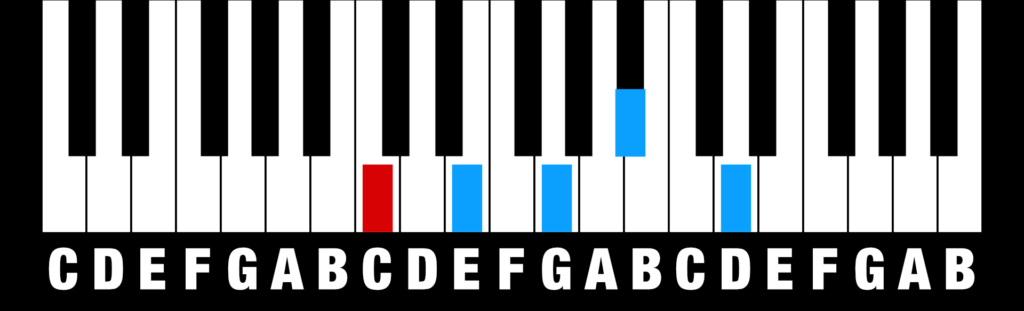### Major 9th (Cmaj9) = R + 4 + 3 + 4 + 3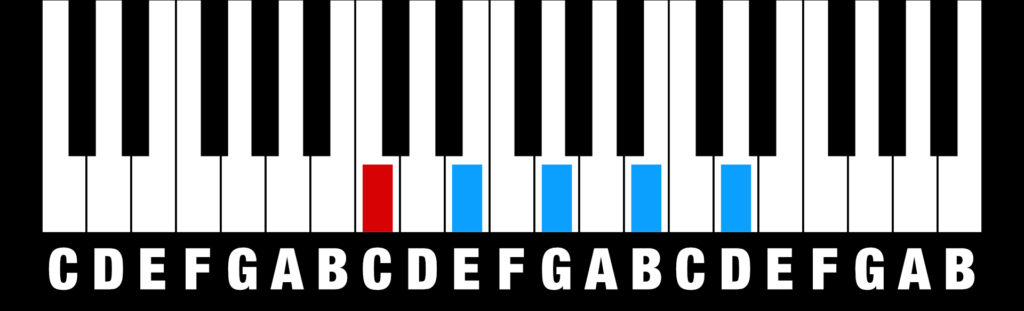### Minor 9th (Cm9) = R + 3 + 4 + 3 + 4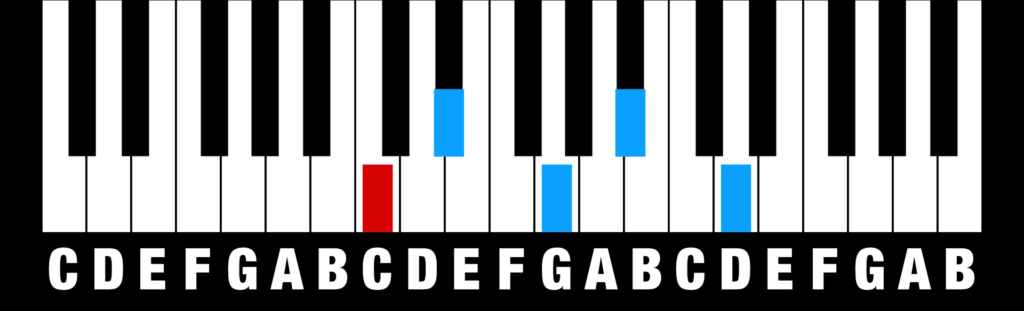### 6/9 chord (C6/9) = R + 4 + 3 + 2 + 5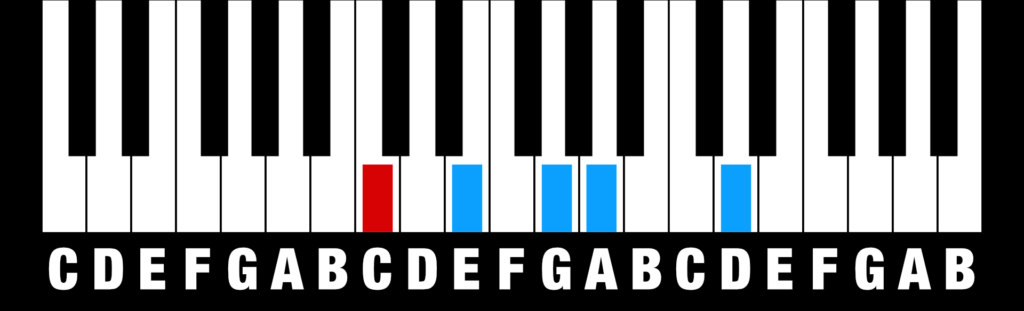### Add 9th (Cadd9) = R + 4 + 3 + 7